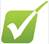Shells, levels and orbitals1)    Line emission spectra's give a unique spectra to each elements.  Explain how they arise.  Use the following terms in your answer: 

Excited        Emit        Energy        Light        Frequency        absorb        Energy level

2)    The picture shows part of a spectra for an element.a)   What do you notice about the spectral lines as you move to the left?  b)   Explain how this spectra has changed the structure of the atom. 

3)    Sketch an energy level diagram showing the first 3 principle quantum numbers and write on how many electrons each energy level can hold. 

4)    The following question is about atomic orbitals:

a)    What is an atomic orbital? 

b)    What is the maximum amount of electrons in an orbital? 

c)    How can 2 electrons occupy the same orbital when they both have a negative charge? 

d)    What can you say about the electron density of an orbital containing 2 electrons rather than 1 electron?